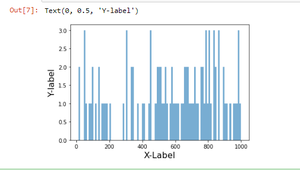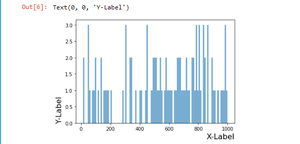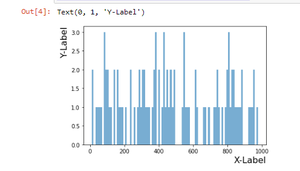Open In App
Related Articles
• Write an Interview Experience

# How To Adjust Position of Axis Labels in Matplotlib?

In this article, we will see how to adjust positions of the x-axis and y-axis labels in Matplotlib which is a library for plotting in python language. By default, these labels are placed in the middle, but we can alter these positions using the “loc” parameter in set_xlabel and set_ylabel function of matplotlib.

Note: “loc” parameter is only available in Matplotlib version 3.3.0 onwards.

Let’s understand with step wise:

Step 1:

First, let’s import all the required libraries.

## Python3

 `import` `matplotlib.pyplot as plt``import` `numpy as np`

Step 2:

Now we will create fake data using the NumPy library. Here we are using the sample sub-module from the random module to create a dataset of random values.

## Python3

 `from` `random ``import` `sample``data ``=` `sample(``range``(``1``, ``1000``), ``100``)`

Step 3:

Now we have created data let’s plot this data using the default options of matplotlib and then start experimenting with its positions. We can clearly see that these labels are in the center by default. The bins parameter tells you the number of bins that your data will be divided into. Matplotlib allows you to adjust the transparency of a graph plot using the alpha attribute. By default, alpha=1

## Python3

 `fig, ax ``=` `plt.subplots() ``ax.hist( data, bins ``=` `100``, alpha ``=` `0.6``) ``ax.set_xlabel(``"X-Label"` `, fontsize ``=` `16``)``ax.set_ylabel(``"Y-label"` `, fontsize ``=` `16``)`

Output:The default position of labels

## Changing the positions of labels using the loc parameter

Here we will move the y-label to the bottom and x-label to the extreme right using the loc parameter.

## Python3

 `fig, ax ``=` `plt.subplots() ``ax.hist( data, bins ``=` `100``, alpha ``=` `0.6``) ``ax.set_xlabel(``"X-Label"``,``              ``fontsize ``=` `16``, loc ``=` `"right"``)`` ` `ax.set_ylabel(``"Y-Label"``, ``              ``fontsize ``=` `16``, loc ``=` `"bottom"``)`

Output:Y-label to the bottom and X-label to extreme right

Let’s take another example here we will move the y-label to the top.

## Python3

 `fig, ax ``=` `plt.subplots() ``ax.hist( data, bins ``=` `100``, alpha ``=` `0.6``) ``ax.set_xlabel(``"X-Label"``, ``              ``fontsize ``=` `16``, loc ``=` `"right"``)`` ` `ax.set_ylabel(``"Y-Label"``, ``              ``fontsize ``=` `16``, loc ``=` `"top"``)`

Output:Y-label to the top and X-label to extreme right